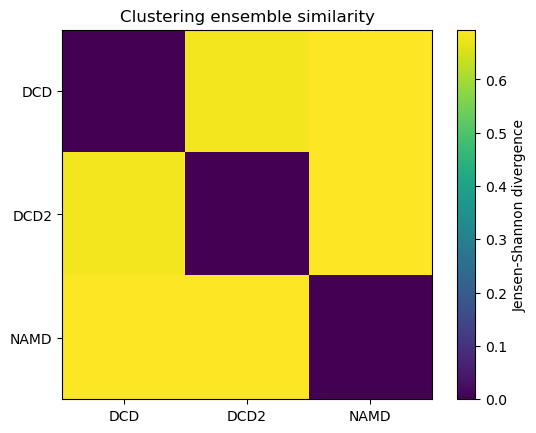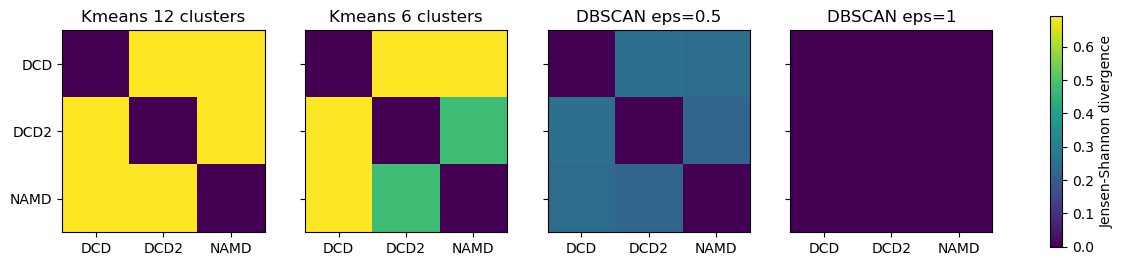# Calculating the Clustering Ensemble Similarity between ensembles

Here we compare the conformational ensembles of proteins in three trajectories, using the clustering ensemble similarity method.

Last updated: December 2022 with MDAnalysis 2.4.0-dev0

Minimum version of MDAnalysis: 1.0.0

Packages required:

Optional packages for visualisation:

Note

The metrics and methods in the encore module are from ([TPB+15]). Please cite them when using the MDAnalysis.analysis.encore module in published work.

:

import numpy as np
import matplotlib.pyplot as plt
%matplotlib inline

import MDAnalysis as mda
from MDAnalysis.tests.datafiles import (PSF, DCD, DCD2, GRO, XTC,
PSF_NAMD_GBIS, DCD_NAMD_GBIS)
from MDAnalysis.analysis import encore
from MDAnalysis.analysis.encore.clustering import ClusteringMethod as clm


The test files we will be working with here feature adenylate kinase (AdK), a phosophotransferase enzyme. ([BDPW09])

:

u1 = mda.Universe(PSF, DCD)
u2 = mda.Universe(PSF, DCD2)
u3 = mda.Universe(PSF_NAMD_GBIS, DCD_NAMD_GBIS)

labels = ['DCD', 'DCD2', 'NAMD']

/home/pbarletta/mambaforge/envs/mda-user-guide/lib/python3.9/site-packages/MDAnalysis/coordinates/DCD.py:165: DeprecationWarning: DCDReader currently makes independent timesteps by copying self.ts while other readers update self.ts inplace. This behaviour will be changed in 3.0 to be the same as other readers


The trajectories can have different lengths, as seen below.

:

print(len(u1.trajectory), len(u2.trajectory), len(u3.trajectory))

98 102 100


## Calculating clustering similarity with default settings

The clustering ensemble similarity method (ces, API docs here) combines every trajectory into a whole space of conformations, and then uses a user-specified clustering_method to partition this into clusters. The population of each trajectory ensemble within each cluster is taken as a probability density function.

The similarity of each probability density function is compared using the Jensen-Shannon divergence. This divergence has an upper bound of $$\ln{(2)}$$, representing no similarity between the ensembles, and a lower bound of 0.0, representing identical conformational ensembles.

You do not need to align your trajectories, as the function will align it for you (along your selection atoms, which are select='name CA' by default).

:

ces0, details0 = encore.ces([u1, u2, u3])


encore.ces returns two outputs. ces0 is the similarity matrix for the ensemble of trajectories.

:

ces0

:

array([[0.        , 0.68070702, 0.69314718],
[0.68070702, 0.        , 0.69314718],
[0.69314718, 0.69314718, 0.        ]])


details0 contains the calculated clusters as a encore.clustering.ClusterCollection.ClusterCollection.

:

cluster_collection = details0['clustering']
print(type(cluster_collection))
print('We have found {} clusters'.format(len(cluster_collection)))

<class 'MDAnalysis.analysis.encore.clustering.ClusterCollection.ClusterCollection'>
We have found 49 clusters


We can access each Cluster at cluster_collection.clusters. For example, the first one has these elements:

:

first_cluster = cluster_collection.clusters
first_cluster

:

<Cluster with 5 elements, centroid=1, id=0>

:

first_cluster.elements

:

array([ 0,  1,  2,  3, 98])


Each cluster has an ID number and a centroid conformation.

:

print('The ID of this cluster is:', first_cluster.id)
print('The centroid is', first_cluster.centroid)

The ID of this cluster is: 0
The centroid is 1


### Plotting

:

fig0, ax0 = plt.subplots()
im0 = plt.imshow(ces0, vmax=np.log(2), vmin=0)
plt.xticks(np.arange(3), labels)
plt.yticks(np.arange(3), labels)
plt.title('Clustering ensemble similarity')
cbar0 = fig0.colorbar(im0)
cbar0.set_label('Jensen-Shannon divergence');## Calculating clustering similarity with one method

Clustering methods should be subclasses of analysis.encore.clustering.ClusteringMethod, initialised with your chosen parameters. Below, we set up an affinity progragation scheme, which uses message-passing to choose a number of ‘exemplar’ points to represent the data and updates these points until they converge. The preference parameter controls how many exemplars are used – a higher value results in more clusters, while a lower value results in fewer clusters. The damping factor damps the message passing to avoid numerical oscillations. (See the scikit-learn user guide for more information.)

The other keyword arguments control when to stop clustering. Adding noise to the data can also avoid numerical oscillations.

:

clustering_method = clm.AffinityPropagationNative(preference=-1.0,
damping=0.9,
max_iter=200,
convergence_iter=30,


By default, MDAnalysis will run the job on one core. If it is taking too long and you have the resources, you can increase the number of cores used.

:

ces1, details1 = encore.ces([u1, u2, u3],
select='name CA',
clustering_method=clustering_method,
ncores=4)


### Plotting

:

fig1, ax1 = plt.subplots()
im1 = plt.imshow(ces1, vmax=np.log(2), vmin=0)
plt.xticks(np.arange(3), labels)
plt.yticks(np.arange(3), labels)
plt.title('Clustering ensemble similarity')
cbar1 = fig1.colorbar(im1)
cbar1.set_label('Jensen-Shannon divergence');## Calculating clustering similarity with multiple methods

You may want to try different clustering methods, or use different parameters within the methods. encore.ces allows you to pass a list of clustering_methods to be applied.

Note

To use the other ENCORE methods available, you need to install scikit-learn.

### Trying out different clustering parameters

The KMeans clustering algorithm separates samples into $$n$$ groups of equal variance, with centroids that minimise the inertia. You must choose how many clusters to partition. (See the scikit-learn user guide for more information.)

:

km1 = clm.KMeans(12,  # no. clusters
init = 'k-means++',  # default
algorithm="auto")    # default

km2 = clm.KMeans(6,  # no. clusters
init = 'k-means++',  # default
algorithm="auto")    # default


The DBSCAN algorithm is a density-based clustering method that defines clusters as ‘high density’ areas, separated by low density areas. The parameters min_samples and eps define how dense an area should be to form a cluster. Clusters are defined around core points which have at least min_samples neighbours within a distance of eps. Points that are at least eps in distance from any core point are considered outliers. (See the scikit-learn user guide for more information.)

A higher min_samples or lower eps mean that data points must be more dense to form a cluster. You should consider your eps carefully. In MDAnalysis, eps can be interpreted as the distance between two points in Angstrom.

Note

DBSCAN is an algorithm that can identify outliers, or data points that don’t fit into any cluster. dres() and dres_convergence() treat the outliers as their own cluster. This means that the Jensen-Shannon divergence will be lower than it should be for trajectories that have outliers. Do not use this clustering method unless you are certain that your trajectories will not have outliers.

:

db1 = clm.DBSCAN(eps=0.5,
min_samples=5,
algorithm='auto',
leaf_size=30)

db2 = clm.DBSCAN(eps=1,
min_samples=5,
algorithm='auto',
leaf_size=30)


When we pass a list of clustering methods to encore.ces, the results get saved in ces2 and details2 in order.

:

ces2, details2 = encore.ces([u1, u2, u3],
select='name CA',
clustering_method=[km1, km2, db1, db2],
ncores=4)
print(len(ces2), len(details2['clustering']))

4 4


### Plotting

:

titles = ['Kmeans 12 clusters', 'Kmeans 6 clusters', 'DBSCAN eps=0.5', 'DBSCAN eps=1']
fig2, axes = plt.subplots(1, 4, sharey=True, figsize=(15, 3))
for i, (data, title) in enumerate(zip(ces2, titles)):
imi = axes[i].imshow(data, vmax=np.log(2), vmin=0)
axes[i].set_xticks(np.arange(3))
axes[i].set_xticklabels(labels)
axes[i].set_title(title)
plt.yticks(np.arange(3), labels)
cbar2 = fig2.colorbar(imi, ax=axes.ravel().tolist())
cbar2.set_label('Jensen-Shannon divergence');As can be seen, reducing the number of clusters in the K-means method emphasises that DCD2 is more similar to the NAMD trajectory than DCD. Meanwhile, increasing eps in DBSCAN clearly lowered the density required to form a cluster so much that every trajectory is in the same cluster, and therefore they have identical probability distributions.

:

n_db = len(details2['clustering'][-1])

print('Number of clusters in DBSCAN eps=1: {}'.format(n_db))

Number of clusters in DBSCAN eps=1: 1


## Estimating the error in a clustering ensemble similarity analysis

encore.ces also allows for error estimation using a bootstrapping method. This returns the average Jensen-Shannon divergence, and standard deviation over the samples.

:

avgs, stds = encore.ces([u1, u2, u3],
select='name CA',
clustering_method=clustering_method,
estimate_error=True,
ncores=4)

:

avgs

:

array([[0.        , 0.68682809, 0.69314718],
[0.68682809, 0.        , 0.69314718],
[0.69314718, 0.69314718, 0.        ]])

:

stds

:

array([[0.00000000e+00, 5.26432545e-03, 7.02166694e-17],
[5.26432545e-03, 0.00000000e+00, 8.59975057e-17],
[7.02166694e-17, 8.59975057e-17, 0.00000000e+00]])